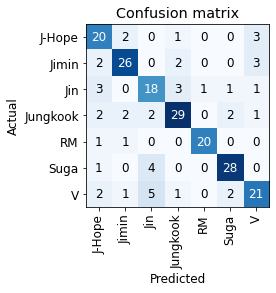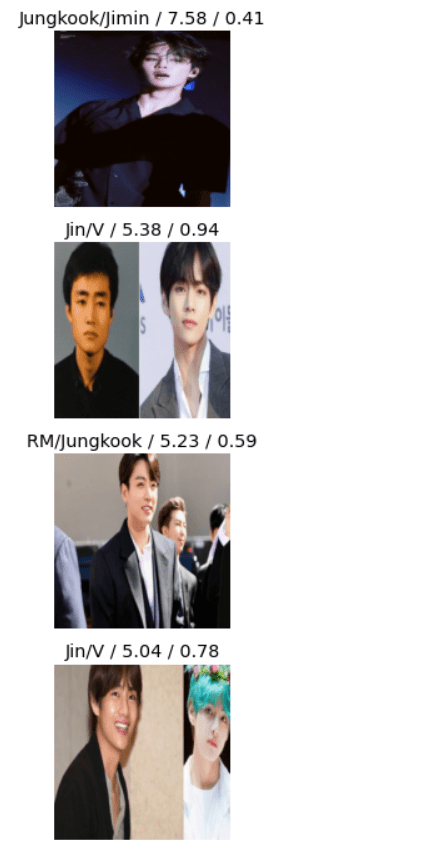Open in App
Not now

# Identify Members of BTS — An Image Classifier

• Last Updated : 27 Sep, 2021

BTS is an eminent K-Pop band comprising of 7 members. This article looks at an image classifier that would recognize the name of the band member from a picture. The image classifier would be built using fastai. It is a deep learning library that aims to democratize deep learning. It is built on top of PyTorch, and has plethora of models with optimized weights that are ready-to-use. The application would be hosted on Binder, and the end product would look like this:

## Preparing the Dataset

As is the case with any image classifier, the model needs to be trained on a dataset from which it can infer and extract the features corresponding to a particular category. The BTS Image Classifier would contain 7 categories (total number of members). The dataset can be prepared by manually collecting images of different members and then clubbing them in a folder of that category. In order to fasten this process, a Python script can be employed to create the dataset. The script would fetch images from Google Image Search. (Disclaimer: Using these images may lead to a copyright violation so proceed at your own risk).

A folder called simple_images would appear at the location where the script is present. Inside the simple_images folder, the folders corresponding to each of the seven members with 150 images would be present.

It is time to code the classifier. It is recommended to use Google Collab (the GPU would come in handy while training) and have the dataset uploaded to Google Drive.

## Python3

 `# Import fastbook ` `from` `fastbook ``import` `*` `from` `fastai.vision.widgets ``import` `*` `from` `google.colab ``import` `drive  ` `drive.mount(``'/content/drive'``) ` ` `  `import` `fastbook ` `fastbook.setup_book() ` ` `  ` `  ` `  `class` `DataLoaders(``GetAttr``): ` `    ``def` `__init__(``self``, ``*``loaders): ``self``.loaders ``=` `loaders ` ` `  `    ``def` `__getitem__(``self``, i): ``return` `self``.loaders[i] ` `    ``train, valid ``=` `add_props(``lambda` `i, ``self``: ``self``[i]) `

DataLoaders is a class that is responsible for providing the valid and train dataset to the model.

## Python3

 `# Import the required function to download from the Simple Image Download library. ` `from` `simple_image_download ``import` `simple_image_download as simp ` `# Create a response instance. ` `response ``=` `simp.simple_image_download ` `# The following lines would look up Google Images and download the number of images specified. ` `# The first argument is the term to search, and the second argument is the number of images to be downloaded. ` `response.download(``'BTS Jin'``, ``150``) ` `response.download(``'BTS Jimin'``, ``150``) ` `response.download(``'BTS RM'``, ``150``) ` `response.download(``'BTS J-Hope'``, ``150``) ` `response.download(``'BTS Suga'``, ``150``) ` `response.download(``'BTS Jungkook'``, ``150``) `

## Cleaning the Data

The images that have been downloaded may not be of the same dimensions. It is preferred to have all the images in the dataset of uniform dimensionality. The fastai library has a function for this:

## Python3

 `bts ``=` `bts.new( ` `    ``item_tfms``=``RandomResizedCrop(``224``, min_scale``=``0.5``), ` `    ``batch_tfms``=``aug_transforms()) ` `dls ``=` `bts.dataloaders(path)`

All the images are resized to 224 x 224 which is a standard size for images in the training dataset.

## Creating the Model

It is time to create the Learner. The Learner is the model that is going to learn from the dataset that is provided. It will then be able to predict the output (independent variable) when supplied with an image that was not a part of the training set. The Learner that is used here is called ‘Resnet18’. It is already pretrained, meaning the weights are tweaked such that the model should be able to reasonable predictions without further tweaks. This idea is called transfer learning

## Python3

 `learn ``=` `cnn_learner(dls, resnet18, metrics``=``error_rate) ` `learn.fine_tune(``8``)`

fine_tune(8) means the learning takes place for 8 epochs. This number can be played with. The tradeoff between accuracy and the computation power/time would be something to consider.

The model is now trained, and the result can be visualized by looking at the confusion matrix.

## Python3

 `interp ``=` `ClassificationInterpretation.from_learner(learn) ` `interp.plot_confusion_matrix()`Ideally, only the diagonal elements of the confusion matrix should be non-zero. It can be seen that in the model’s predictions, there are some misclassifications present.

The images with the top losses can be seen. These are usually the images which the model predicts incorrectly with great certainty or correctly with less certainty.

## Python3

 `interp.plot_top_losses(``5``, nrows``=``5``)`## Deploying the Model

The model would be deployed using Binder. The GitHub URL of the notebook needs to be pasted. Firstly, the model needs to be exported, and a file with the extension .pkl  needs to be generated.

## Python3

 `learn.export() ` `path ``=` `Path() ` `path.ls(file_exts``=``'.pkl'``)`

Visit the website for Binder. Paste the URL of the GitHub repository, containing the notebook and .pkl file. In the ‘URL to open’ blank, enter the (GitHub) URL of the notebook. Click on ‘launch’, and after a few minutes, the web-application would be ready to be used.

Note: This image classifier was first taught in the Fast.AI Deep Learning course, lecture 2.

My Personal Notes arrow_drop_up
Related Articles